# Maharashtra Board 12th Commerce Maths Solutions Chapter 2 Matrices Ex 2.3

Balbharati Maharashtra State Board 12th Commerce Maths Solution Book Pdf Chapter 2 Matrices Ex 2.3 Questions and Answers.

## Maharashtra State Board 12th Commerce Maths Solutions Chapter 2 Matrices Ex 2.3

Question 1.
Evaluate:
(i) $$\left[\begin{array}{l} 3 \\ 2 \\ 1 \end{array}\right]\left[\begin{array}{lll} 2 & -4 & 3 \end{array}\right]$$
Solution:
$$\left[\begin{array}{l} 3 \\ 2 \\ 1 \end{array}\right]\left[\begin{array}{lll} 2 & -4 & 3 \end{array}\right]$$ = $$\left[\begin{array}{rrr} 6 & -12 & 9 \\ 4 & -8 & 6 \\ 2 & -4 & 3 \end{array}\right]$$

(ii) $$\left[\begin{array}{lll} 2 & -1 & 3 \end{array}\right]\left[\begin{array}{l} 4 \\ 3 \\ 1 \end{array}\right]$$
Solution:
$$\left[\begin{array}{lll} 2 & -1 & 3 \end{array}\right]\left[\begin{array}{l} 4 \\ 3 \\ 1 \end{array}\right]$$ = [8 – 3 + 3] =Question 2.
If A = $$\left[\begin{array}{ccc} -1 & 1 & 1 \\ 2 & 3 & 0 \\ 1 & -3 & 1 \end{array}\right]$$, B = $$\left[\begin{array}{lll} 2 & 1 & 4 \\ 3 & 0 & 2 \\ 1 & 2 & 1 \end{array}\right]$$. State whether AB = BA? Justify your answer.
Solution: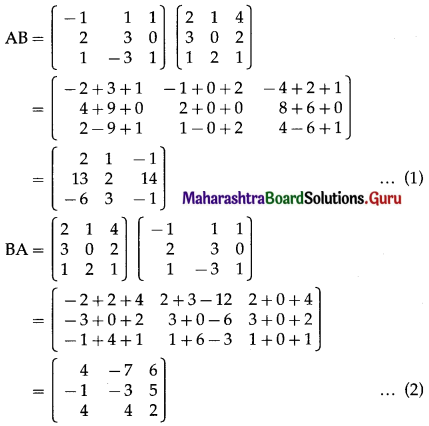From (1) and (2), AB ≠ BA.

Question 3.
Show that AB = BA, where A = $$\left[\begin{array}{lll} -2 & 3 & -1 \\ -1 & 2 & -1 \\ -6 & 9 & -4 \end{array}\right]$$, B = $$\left[\begin{array}{rrr} 1 & 3 & -1 \\ 2 & 2 & -1 \\ 3 & 0 & -1 \end{array}\right]$$
Solution: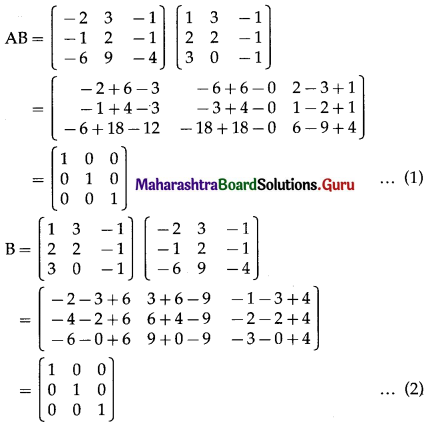From (1) and (2), AB = BA.

Question 4.
Verify A(BC) = (AB)C, if A = $$\left[\begin{array}{lll} 1 & 0 & 1 \\ 2 & 3 & 0 \\ 0 & 4 & 5 \end{array}\right]$$, B = $$\left[\begin{array}{cc} 2 & -2 \\ -1 & 1 \\ 0 & 3 \end{array}\right]$$, and C = $$\left[\begin{array}{rrr} 3 & 2 & -1 \\ 2 & 0 & -2 \end{array}\right]$$
Solution: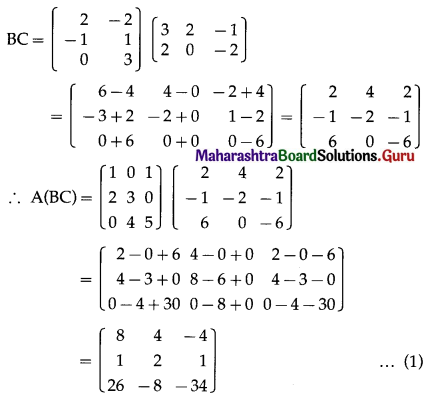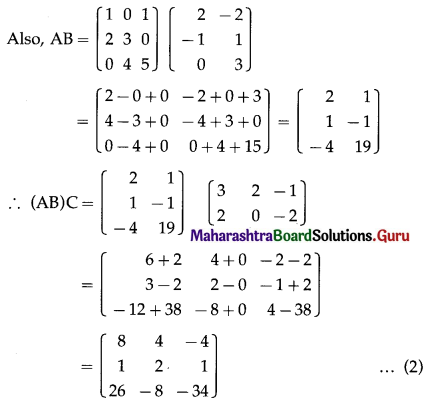From (1) and (2), A(BC) = (AB)C.Question 5.
Verify that A(B + C) = AB + AC, if A = $$\left[\begin{array}{cc} 4 & -2 \\ 2 & 3 \end{array}\right]$$, B = $$\left[\begin{array}{cc} -1 & 1 \\ 3 & -2 \end{array}\right]$$ and C = $$\left[\begin{array}{cc} 4 & 1 \\ 2 & -1 \end{array}\right]$$
Solution: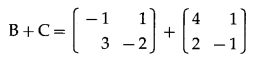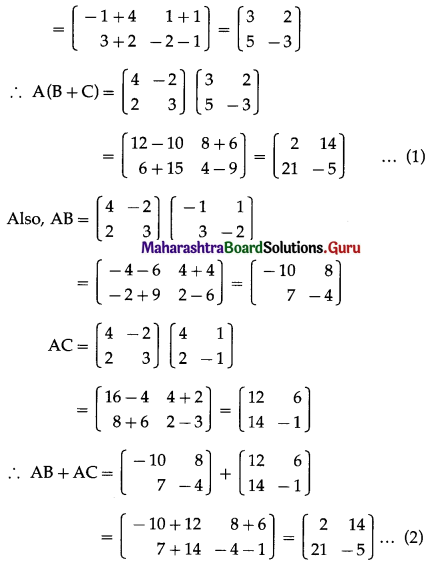From (1) and (2), A(B + C) = AB + AC.

Question 6.
If A = $$\left[\begin{array}{ccc} 4 & 3 & 2 \\ -1 & 2 & 0 \end{array}\right]$$, B = $$\left[\begin{array}{cc} 1 & 2 \\ -1 & 0 \\ 1 & -2 \end{array}\right]$$, show that matrix AB is non-singular.
Solution: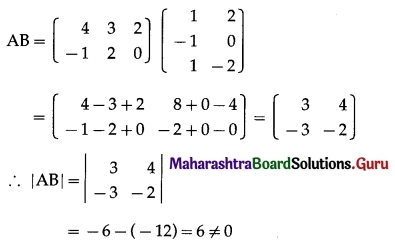Hence, AB is a non-singular matrix.

Question 7.
If A + I = $$\left[\begin{array}{ccc} 1 & 2 & 0 \\ 5 & 4 & 2 \\ 0 & 7 & -3 \end{array}\right]$$, find the product (A + I)(A – I).
Solution: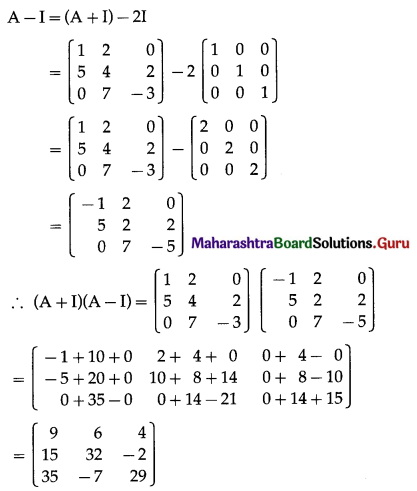Question 8.
If A = $$\left[\begin{array}{lll} 1 & 2 & 2 \\ 2 & 1 & 2 \\ 2 & 2 & 1 \end{array}\right]$$, show that A2 – 4A is a scalar matrix.
Solution: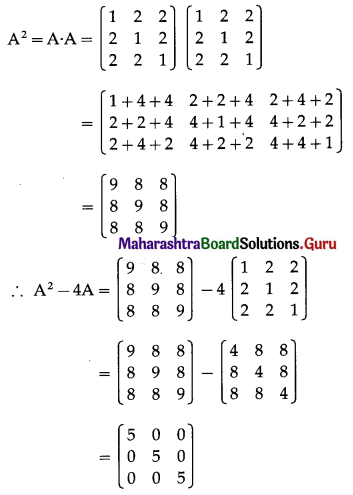which is a scalar matrix.

Question 9.
If A = $$\left[\begin{array}{cc} 1 & 0 \\ -1 & 7 \end{array}\right]$$, find k so that A2 – 8A – kI = O, where I is a 2 × 2 unit matrix and O is null matrix of order 2.
Solution: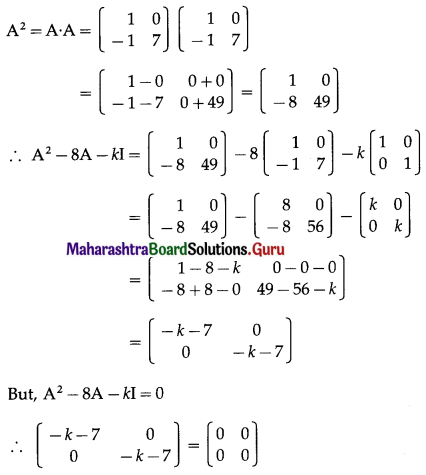By equality of matrices,
-k – 7 = 0
∴ k = -7.

Question 10.
If A = $$\left[\begin{array}{cc} 3 & 1 \\ -1 & 2 \end{array}\right]$$, prove that A2 – 5A + 7I = 0, where I is a 2 × 2 unit matrix.
Solution: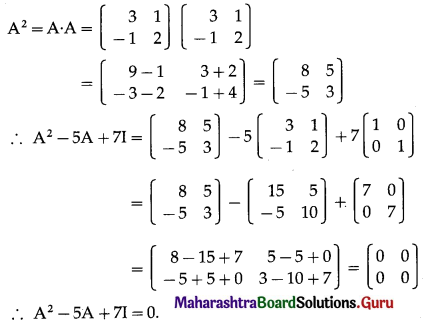Question 11.
If A = $$\left[\begin{array}{cc} 1 & 2 \\ -1 & -2 \end{array}\right]$$, B = $$\left[\begin{array}{cc} 2 & a \\ -1 & b \end{array}\right]$$ and if(A + B)2 = A2 + B2, find values of a and b.
Solution:
(A + B)2 = A2 + B2
∴ (A + B)(A + B) = A2 + B2
∴ A2 + AB + BA + B2 = A2 + B2
∴ AB + BA = 0
∴ AB = -BA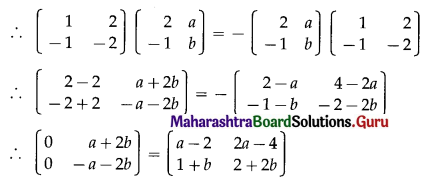By the equality of matrices, we get
0 = a – 2 ……..(1)
0 = 1 + b ……..(2)
a + 2b = 2a – 4 ……..(3)
-a – 2b = 2 + 2b ……..(4)
From equations (1) and (2), we get
a = 2 and b = -1
The values of a and b satisfy equations (3) and (4) also.
Hence, a = 2 and b = -1.Question 12.
Find k, if A = $$\left[\begin{array}{ll} 3 & -2 \\ 4 & -2 \end{array}\right]$$ and A2 = kA – 2I.
Solution: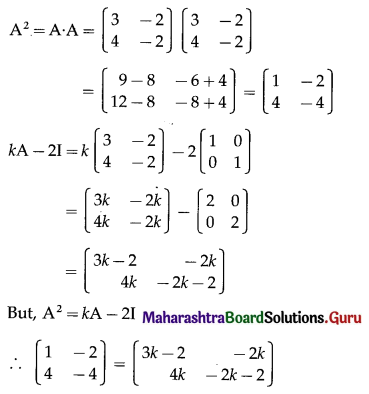By equality of matrices,
1 = 3k – 2 ……..(1)
-2 = -2k ……..(2)
4 = 4k ……..(3)
-4 = -2k – 2 ……..(4)
From (2), k = 1.
k = 1 also satisfies equation (1), (3) and (4).
Hence, k = 1.

Question 13.
Find x and y, if $$\left\{4\left[\begin{array}{ccc} 2 & -1 & 3 \\ 1 & 0 & 2 \end{array}\right]-\left[\begin{array}{ccc} 3 & -3 & 4 \\ 2 & 1 & 1 \end{array}\right]\right\}\left[\begin{array}{c} 2 \\ -1 \\ 1 \end{array}\right]=\left[\begin{array}{c} x \\ y \end{array}\right]$$
Solution: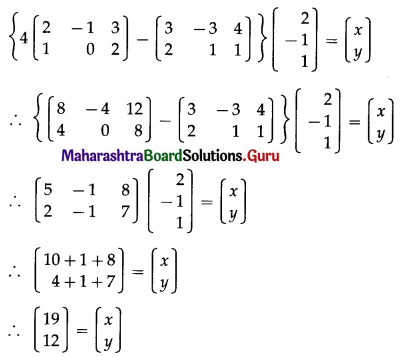By equality of matrices,
x = 19 and y = 12.Question 14.
Find x, y, z, if $$\left\{3\left[\begin{array}{ll} 2 & 0 \\ 0 & 2 \\ 2 & 2 \end{array}\right]-4\left[\begin{array}{cc} 1 & 1 \\ -1 & 2 \\ 3 & 1 \end{array}\right]\right\}\left[\begin{array}{l} 1 \\ 2 \end{array}\right]=\left[\begin{array}{c} x-3 \\ y-1 \\ 2 z \end{array}\right]$$
Solution: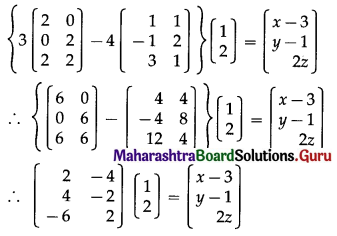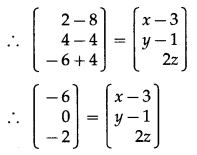By equality of matrices,
-6 = x – 3, 0 = y – 1 and -2 = 2z
∴ x = -3, y = 1 and z = -1.Question 15.
Jay and Ram are two friends. Jay wants to buy 4 pens and 8 notebooks. Ram wants to buy 5 pens and 12 notebooks. The price of one pen and one notebook was ₹ 6 and ₹ 10 respectively. Using matrix multiplication, find the amount each one of them requires for buying the pens and notebooks.
Solution:
The given data can be written in matrix form as:
Number of Pens and Notebooks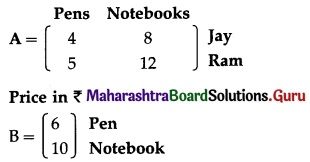For finding the amount each one of them requires to buy the pens and notebook, we require the multiplication of the two matrices A and B.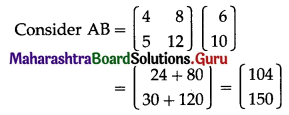Hence, Jay requires ₹ 104 and Ram requires ₹ 150 to buy the pens and notebooks.